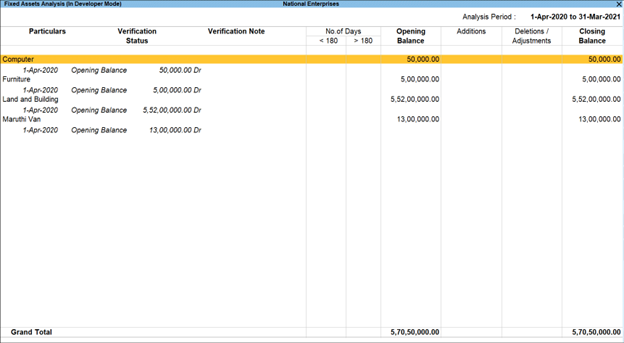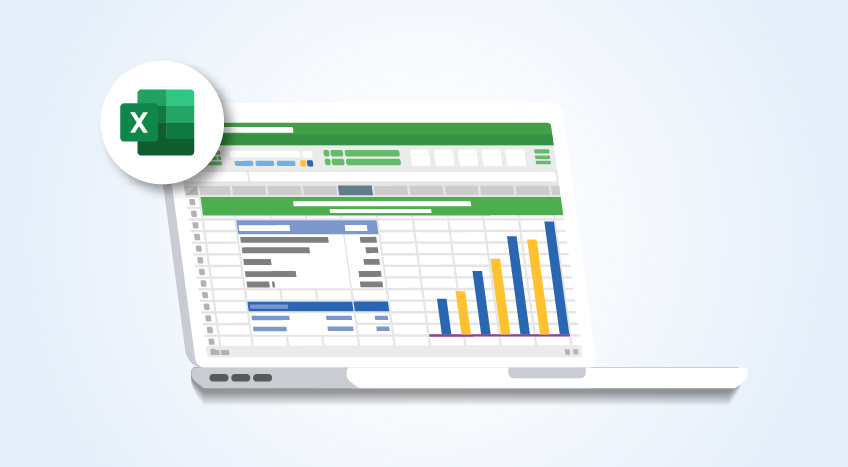# What is Straight Line Depreciation & How To Calculate Straight Line Depreciation?

##### Tally Solutions|Updated on: June 17, 2022

In accounting, various principles are used to match sales and expenses. This is required for all the items that the company purchases and uses. Unless the assets are properly expensed, the usage and loss of value of the asset over time will not be accurately reflected in the books of accounts. So extending the expense for an asset purchase over the period of its usage is the method followed. This is called depreciation. One of the most straightforward ways to calculate depreciation is the straight line basis. It divides the expensed amount equally over the usage period. It is simple to calculate and very easy to understand. Amortization is the method used to expense intangible assets, while deprecation is the method to expense tangible assets.

## What is straight line basis?

The straight line basis or straight line method of depreciation is used to calculate depreciation and amortization. It is a simple way to calculate the value that an asset loses over time. Every asset is purchased at a cost and may have a salvage value at the end of its life. The difference between the asset’s purchase cost and the salvage value is divided by the number of usable years you expect to use it. This number is the straight line basis depreciation value which is used to expense an asset over a longer period of time. The straight line basis is commonly used simply because it is very easy to understand and calculate. It does have its own drawbacks but remains popular despite them since other methods accelerate the schedule of depreciation.

Straight line depreciation assumes that an asset wears out at the same rate over time. The other methods of depreciation work on the principle that things wear out at different rates at different periods of time. Some of these methods are the sum of years method, the double-declining balance method, and the unit-of-production method. They reflect the fact that most assets, such as vehicles, machinery, and electronics, lose most of their value in the first few years of their use. But, the straight line method is the simplest.

Application of straight line basis

• Straight line basis is used when you need to expense an asset in a simple and easy-to-calculate manner. If you want to write off an asset faster, the other accelerated methods of calculating depreciation are better. It is best to use the technique that is required by authorities or that suits accounting and industry best practices.
• The value of the business can only be accurately calculated when the assets that are owned are accurately expensed over time. Depreciation contributes to the expenses in companies that use a lot of expensive equipment over long periods of time.
• The calculation of depreciation is essential for a balance sheet or income statement to accurately reflect the resources that have been used to generate income. The straight line method allows accountants to do that quickly and simply.

## Calculating straight line basis

The straight line method of depreciation assumes that an asset decreases in value at a steady and even rate over its usable life. The value that it loses is calculated as the difference between the salvage value and purchase value of the asset. This is then divided by the useful life of the asset. The formula used for the calculation is:

Straight Line Basis = (Purchase Price of Asset - Salvage Value) / Estimated Useful Life of Asset

The unit-of-production method is also similar to the straight-line depreciation but uses units of production as a measure instead of a monetary value. These units of production are particular to the industry and the item that is being produced using that asset. This method is based on the principle that assets wear out based on usage rather than the number of years of use. This calculation requires the accountant to know the number of units the asset produced in that year and the total number of units it is expected to produce over its lifetime. The formula used is:

Annual Depreciation In Number Of Units = (Purchase Price - Salvage Value) X ( Number Of Units Produced That Year) / Total Number Of Units Expected Over Its Lifetime

The unit of production method is used for tools and equipment that wear out with use rather than time.

## Example of straight line basis

Company A buys a machine that costs \$9000 and expects it to have a usable life of 10 years and a salvage value of \$500. To calculate the straight line basis or straight line depreciation, we use the following formula:

Straight Line Basis = (Purchase Price of Asset - Salvage Value) / Estimated Useful Life of Asset

Straight Line Basis = (\$9000- \$500) / 10 = \$8500/10 = \$850

So, every year, the company’s accountants will expense the straight line basis amount of \$850 for that asset until its value reaches the expected salvage value of \$500.

## How do you calculate straight line depreciation?

Calculating the straight line basis requires fewer numbers than the other methods of calculation. You need:

• The total purchase price of the asset, which includes shipping, taxes, and other related costs
• The scrap or salvage value expected at the end of its usable life
• The expected usable life of the asset

The first step in the straight line method of depreciation is to subtract the salvage value from the cost, which amounts to the total depreciation. This is then divided by the asset's useful life to obtain the annual depreciation value. The formula is:

Annual Depreciation = (Purchase Price - Salvage Value) / Useful Life

This is the value that must be subtracted from the asset's value every year until it reaches the salvage value. The remaining value of the asset after this annual subtraction is called the book value and it reflects the current value of the asset after its depreciation over time. The local rules and regulations may define the useful life of every asset.

Straight line depreciation is easy to explain and easy to understand. Accountants also prefer this method as it is simple and easy to use with minimal information. There are minimal errors in the usage of this method as the amount of depreciation deducted every year is the same.

The simplicity of the straight line depreciation method is also one of its disadvantages. The estimate of the life is usually guesswork, and the asset may become obsolete or unusable sooner than expected.

In reality, many assets lose value quickly in the first few years and then depreciate slowly. The straight line basis does not reflect that accurately. Some assets have higher maintenance expenses as they age, and this is also not included in the straight line method.

## What are realistic assumptions in the straight-line method of depreciation?

The straight line method of depreciation requires the accountant to know the asset's purchase value, salvage value, and usable life. The only number that the accountant is very sure of is the purchase value. The salvage value and the usable life are assumptions. With experience, these assumed numbers are usually right, but when they are wrong the result could be a rather costly mistake. The assumption that assets decrease at a steady rate over the years may also be wrong. Some assets decline in value much quicker. Machinery and equipment that wear out with use may lose value quicker in periods when they are used more. This is not factored in the straight line method of depreciation.

## What is straight line amortization?

Straight line amortization is similar to straight line depreciation. The main difference is that amortization is the term applied to the loss of value of an intangible asset such as intellectual property.

## Easy depreciation calculations with Tally

The year-end preparation of financial reports and statements is quick and easy with TallyPrime. Amortization and depreciation are calculated accurately, and the appropriate entries are reflected in the relevant accounts. Bring the power of intelligent business accounting management to your business and see the difference today!The Periodic Breakup Of Depreciation

Language

#### Latest Blogs